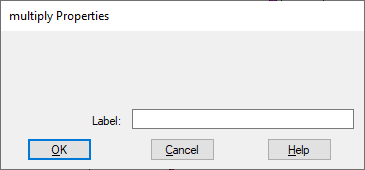## multiply

Block Category: Matrix Operation

Description: The multiply block performs a matrix multiplication. The multiply block accepts two vector inputs and produces one vector output.

To multiply two or more scalars, use the * block. To perform a single value summation of an element-by-element multiply of two vectors, use the dotproduct block.Label: Indicates a user-defined block label that appears when View > Block Labels is activated.